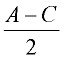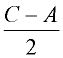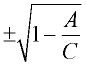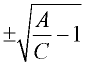Literal Equations - Practice Terms of Use    Contact Person: Donna RobertsDirections: Read carefully to determine the variable for which you are to solve.

1.
If k = am + 3mx, the value of m in terms of a, k and x can be expressed as: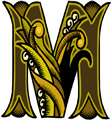Choose: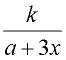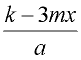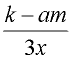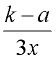2.
If ba - cd = b, which expression represents a?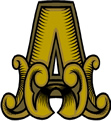Choose: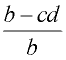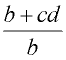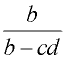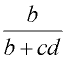3.
If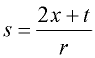, then t equals: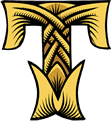Choose: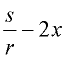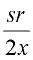sr + 2x sr - 2x

4.
The volume of a pyramid is given by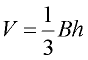.
What is h expressed in terms of B and V ?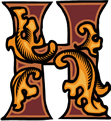Choose: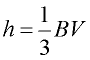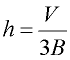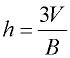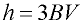5.
For the equation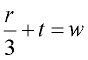, express r in terms of
t and w.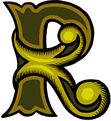Choose:
 r = 3w - t r = 3tw r = 3(w - t)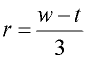6.
If P = Q2O, express O in terms of P and Q.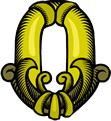Choose: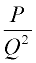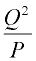P - Q2 P + Q2

7.
Given: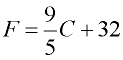.    Solve for C.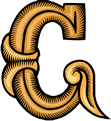Choose: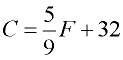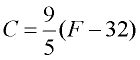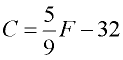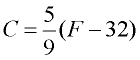8.
A formula is expressed as D = a(2 + kt). Express k in terms of D, a and t.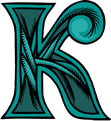Choose: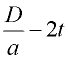D - 2at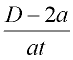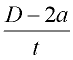9.
If A = C(1 - S2), express S in terms of A and C.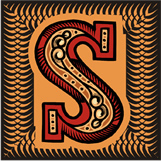Choose: